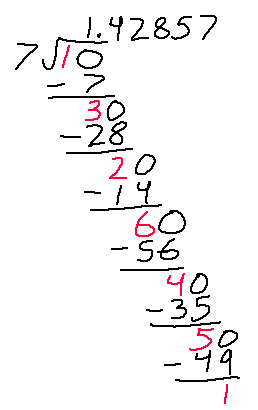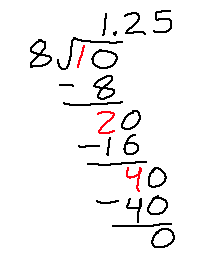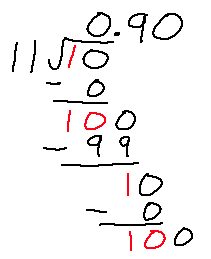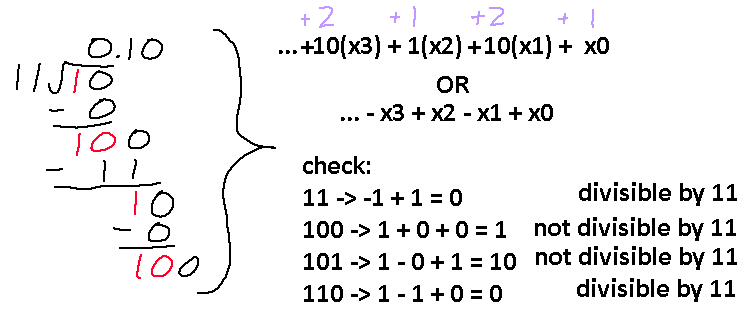# Exploring Divisibility Formulas

Here’s another trick that you may have learned in your time at school; how to tell if a number is divisible by 9? The easy way for large numbers is you add up each digit in the number. If the result you have is not a single digit, then add those digits up until you end up with a single digit. If the final result is 9, then the original number is divisible by 9!

Another popular one I remember is divisibility by 3. Do the same recursive digit summing technique, and if the final number is a multiple of 3, then the original number is a multiple of 3. Naturally, the next question to ask is can you check if a number is divisible by more than just 3 or 9? Obviously there is, or this post wouldn’t have existed.

Let’s start with 2. Divisibility by 2 is easy: if the number is even, it is divisible by 2. What does this mean in terms of adding up digits? Well, it turns out that for any number you only need to look at the last digit, unlike the case with 3 and 9. The valid ending digits are 0, 2, 4, 6, and 8. There is another number that has this really nice property, and that is 5. Any number that ends in 0 or 5 is a multiple of 5. Is it strange that 2 and 5 are the ones with this property, or does it have something to do with the base we are working in?….

Unfortunately, intuition can only take us so far. How about 4? Try listing out all the multiples of 4, and you’ll quickly notice that 0, 2, 4, 6, and 8 are also valid ending digits. But, we know that some numbers are divisible by 2 but not by 4. So, merely looking at the last digit isn’t enough. We must also take the second to last digit into account, and actually that is the only extra piece of information we need. This may seem unrelated, but this is because 100 is divisible by 4. If 100 is divisible by 4, then so is 200, 300, 400, etc. Basically what this means is that any digits after the 1’s and 10’s position do not ‘factor’ into this divisibility check. But, simply adding the two digits up will not get you the correct result. What we are looking for is a final digit of 4 or 8, with any other ending meaning the number isn’t divisible by 4.

Let’s take 12 and 16, for instance. Adding the digits up give 3 and 7. Useful if we were doing a divisibility check of 3, but not for 4. However, if we added 1 to both 3 and 7, we get 4 and 8, which shows that 12 and 16 are multiples of 4. What if we got this extra “1” from doubling the value in the tens place? Then 12 is 1(2) + 2 = 4, and 24 is 2(2) + 4 = 8. Doubling the tens place value and adding it to the ones place value is the correct check for divisibility by 4. Try it yourself using any number!

There is a simple rule to follow. To check if some number is divisible by N, the final result of doing some summing algorithm must also be divisible by N. For 4, the summing algorithm is 2(tens place) + 1(ones place) , and if the result is also a multiple of 4, then the original number is divisible by 4. For 3, it is summing all digits without modifying their values, and making sure the result ends in 3, 6, or 9. With 9, it’s the same algorithm but the result must only end in 9. By understanding this simple rule, we now have a way to slowly build up to finding a summing algorithm of any number.

Seven is our first nasty number, but we can slowly figure out the algorithm by first only considering 2-digit numbers divisible by 7. Here are some: 14, 21, 28, 35, 49, 63, 84, 91. Since we are only worrying about two digits, at most the algorithm needs to consider digits up to the tens place. This is your opportunity to try and find out what summation needs to be done to check divisibility by 7. Give it a try now, because I will give the answer below.

Okay. Turns out that the tens digit needs to be multiplied by 3. For 91, you get 3(9) + 1 = 28, which is 2(3) + 8 = 14, which is 1(4) + 3 = 7. I will now introduce new notation to show the summing algorithm. For 7, the formula is 3(x1) + 1(x0), where x0, x1, x2, etc. is the first, second, third, etc. digit from the right. Just like the 3 check, the 7 check requires adding up all digits, but each digit must be multiplied by a different constant. For 7, the full formula is:

``` …. + 5(x5) + 4(x4) + 6(x3) + 2(x2) + 3(x1) + 1(x0) ```

After the ellipses, the pattern starts again at 1(x6). You can slowly figure this out from the 2-digit formula by only considering 3-digit numbers, using the 3(x1) + 1(x0) formula you already know to try and figure out the multiplier needed for the hundreds digit. It’s important to note that once you hit a multiplier you’ve already seen before (such as 1) then you found the end of the pattern.

There is one last interesting thing to note here before moving on to the general solution. For any digit multiplier, you can add or subtract N from it and you will still get a valid algorithm. The only difference is since now you introduce negatives, the reduced number can become 0 instead of N (or even negative N). If the number is 0 or -N, it is still a valid multiple of N.

For example, we can modify the 3 pattern to something like this: ``` ….. + 1(x4) - 2(x3) - 2(x2) + 1(x1) + 1(x0) ```

I subtracted 3 from the multipliers in x2 and x3 and it’s still a valid algorithm. This becomes useful once larger numbers are introduced since you can sometimes shorten the math required. For example, the 11 pattern will look like this: ``` …. + 1(x4) + 10(x3) + 1(x2) + 10(x1) + 1(x0) ```

If you subtract 11 from those tens then you get the equivalent formula: ``` …. + 1(x4) - 1(x3) + 1(x2) - 1(x1) + 1(x0) ```

Here are some other patterns found using this recursive digit sum:

```            ```
Divisibility by 8: 4(x2) + 2(x1) + 1(x0)
Divisibility by 12: …. + 4(x3) + 4(x2) - 2(x1) + x0
Divisibility by 12: …. - 5(x3) - 5(x2) - 5(x1) + x0
Divisibility by 17: …. + 7(x9) - 1(x8) + 5(x7) - 8(x6) + 6(x5) + 4(x4) - 3(x3) - 2(x2) - 7(x1) + 1(x0)
Divisibility by 25: 10(x1) + 1(x0)

```
```

Well that got complicated quickly, but we are almost done! All of this is a lot of work, but there is a faster way to get these hidden formulas. The answer is long division.

Let’s tackle that 7 divisibility problem again. The divisor is the number we are checking divisibility of, and the dividend is… the base we are working in! Put 10 under the radical and start dividing.Okay, so you’ll basically be dividing this out forever, but look at the numbers we are getting. The seven divisibility formula is this:

``` …. + 5(x5) + 4(x4) + 6(x3) + 2(x2) + 3(x1) + 1(x0) ```

There is a correlation between the multipliers found in this formula and the remainder values that are received from this continuous division. The numbers marked in red match exactly what we have going on here. So the shortcut to getting these multipliers is to pause after every remainder received, and using that value before continuing with the rest of the division. Here are a couple more examples.And since I know you’re dying to know, here is a formula for divisibility of 3, but written in base 2.